# Circular barplot with groups in Matplotlib

This post explains how to build a circular barplot with groups. A gap is added between groups to highlight them. Bars are labeled and group names are annotated. Step-by-step guides are provided.

A circular barplot is a barplot where bars are displayed along a circle instead of a horizontal or vertical line. This page is the continuation of this post on basic circular barplots and aims to teach you how to make a circular barplot with groups.

Let's import the libraries needed and generate the data needed for today's guide.

``````import matplotlib.pyplot as plt
import numpy as np
import pandas as pd``````
``````# Ensures reproducibility of random numbers
rng = np.random.default_rng(123)``````
``````# Build a dataset
df = pd.DataFrame({
"name": [f"item {i}" for i in range(1, 51)],
"value": rng.integers(low=30, high=100, size=50),
"group": ["A"] * 10 + ["B"] * 20 + ["C"] * 12 + ["D"] * 8
})

# Show 3 first rows
name value group
0 item 1 31 A
1 item 2 77 A
2 item 3 71 A

The following is a helper function that given the angle at which the bar is positioned and the offset used in the barchart, determines the rotation and alignment of the labels.

``````def get_label_rotation(angle, offset):
# Rotation must be specified in degrees :(
if angle <= np.pi:
alignment = "right"
rotation = rotation + 180
else:
alignment = "left"
return rotation, alignment``````

And this is the function that actually adds the labels (with `ax.text()`) to the plot:

``````def add_labels(angles, values, labels, offset, ax):

# This is the space between the end of the bar and the label

# Iterate over angles, values, and labels, to add all of them.
for angle, value, label, in zip(angles, values, labels):
angle = angle

# Obtain text rotation and alignment
rotation, alignment = get_label_rotation(angle, offset)

# And finally add the text
ax.text(
x=angle,
s=label,
ha=alignment,
va="center",
rotation=rotation,
rotation_mode="anchor"
) ``````

## Basic circular barplot with labels

Before creating a circular barplot with groups, let's review how to create a circular barplot with labels at the end of each bar. First of all, let's create `ANGLES`, which give the positions where bars are located. The `VALUES` array contains the heights of the bars, and `LABELS` stores the labels.

``````ANGLES = np.linspace(0, 2 * np.pi, len(df), endpoint=False)
VALUES = df["value"].values
LABELS = df["name"].values

# Determine the width of each bar.
# The circumference is '2 * pi', so we divide that total width over the number of bars.
WIDTH = 2 * np.pi / len(VALUES)

# Determines where to place the first bar.
# By default, matplotlib starts at 0 (the first bar is horizontal)
# but here we say we want to start at pi/2 (90 deg)
OFFSET = np.pi / 2

# Initialize Figure and Axis
fig, ax = plt.subplots(figsize=(20, 10), subplot_kw={"projection": "polar"})

# Specify offset
ax.set_theta_offset(OFFSET)

# Set limits for radial (y) axis. The negative lower bound creates the whole in the middle.
ax.set_ylim(-100, 100)

# Remove all spines
ax.set_frame_on(False)

# Remove grid and tick marks
ax.xaxis.grid(False)
ax.yaxis.grid(False)
ax.set_xticks([])
ax.set_yticks([])

ax.bar(
ANGLES, VALUES, width=WIDTH, linewidth=2,
color="#61a4b2", edgecolor="white"
)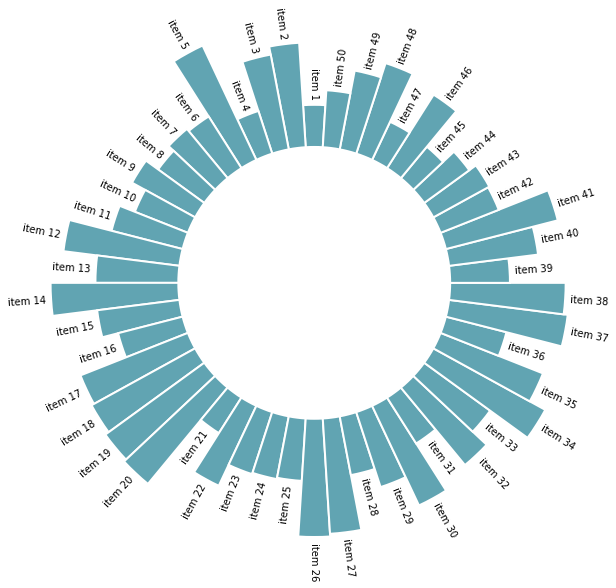## Add a gap in the circle

The next step is to build a circular barplot with a break in the circle. Actually, the approach is just to increase the number of values in `ANGLES`, but leaving some of them unused so it creates the gap.

``````# 3 empty bars are added
ANGLES = np.linspace(0, 2 * np.pi, num=ANGLES_N, endpoint=False)
WIDTH = (2 * np.pi) / len(ANGLES)

# The index contains non-empty bards
IDXS = slice(0, ANGLES_N - PAD)

# The layout customization is the same as above
fig, ax = plt.subplots(figsize=(20, 10), subplot_kw={"projection": "polar"})

ax.set_theta_offset(OFFSET)
ax.set_ylim(-100, 100)
ax.set_frame_on(False)
ax.xaxis.grid(False)
ax.yaxis.grid(False)
ax.set_xticks([])
ax.set_yticks([])

# Add bars, subsetting angles to use only those that correspond to non-empty bars
ax.bar(
ANGLES[IDXS], VALUES, width=WIDTH, color="#61a4b2",
edgecolor="white", linewidth=2
)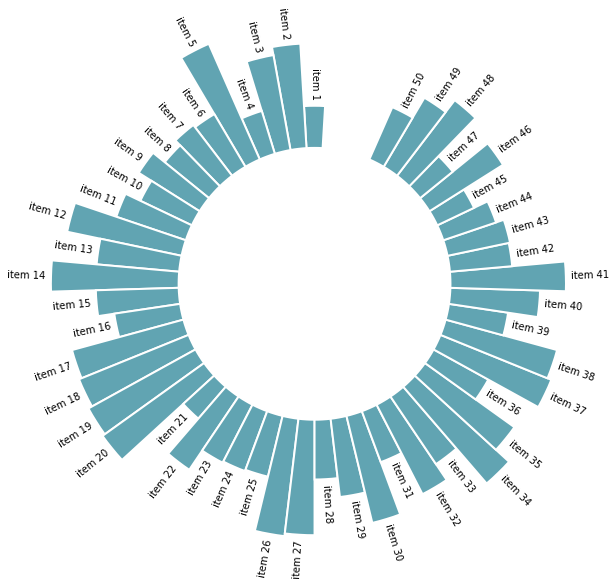## Space between groups

This concept can now be used to add space between each group of the dataset. In this case, `PAD` empty bars are added at the end of each group.

This chart is far more insightful since it allows one to quickly compare the different groups, and to compare the value of items within each group.

``````# Grab the group values
GROUP = df["group"].values

# Add three empty bars to the end of each group
ANGLES_N = len(VALUES) + PAD * len(np.unique(GROUP))
ANGLES = np.linspace(0, 2 * np.pi, num=ANGLES_N, endpoint=False)
WIDTH = (2 * np.pi) / len(ANGLES)

# Obtaining the right indexes is now a little more complicated
offset = 0
IDXS = []
GROUPS_SIZE = [10, 20, 12, 8]
for size in GROUPS_SIZE:

# Same layout as above
fig, ax = plt.subplots(figsize=(20, 10), subplot_kw={"projection": "polar"})

ax.set_theta_offset(OFFSET)
ax.set_ylim(-100, 100)
ax.set_frame_on(False)
ax.xaxis.grid(False)
ax.yaxis.grid(False)
ax.set_xticks([])
ax.set_yticks([])

# Use different colors for each group!
GROUPS_SIZE = [10, 20, 12, 8]
COLORS = [f"C{i}" for i, size in enumerate(GROUPS_SIZE) for _ in range(size)]

# And finally add the bars.
# Note again the `ANGLES[IDXS]` to drop some angles that leave the space between bars.
ax.bar(
ANGLES[IDXS], VALUES, width=WIDTH, color=COLORS,
edgecolor="white", linewidth=2
)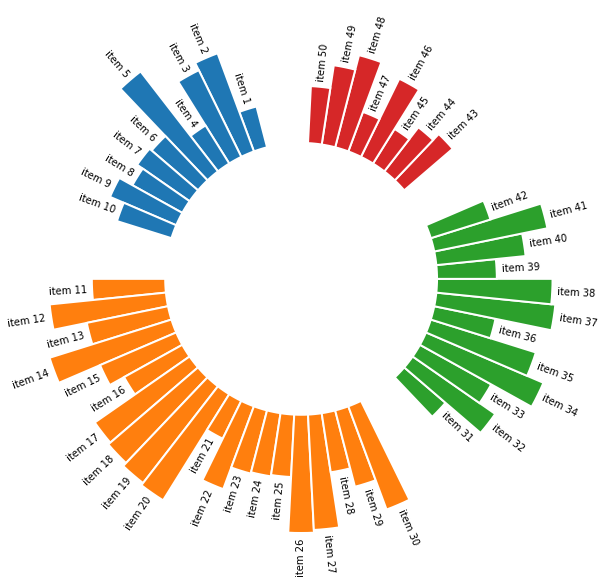## Order bars

Here observations are sorted by bar height within each group. It can be useful if your goal is to understand what are the highest / lowest observations within and across groups.

The method does not modify the code to produce the plot, it only sort values using pandas methods. Basically, you just have to add the following piece of code right after the data frame creation:

``````# Reorder the dataframe
df_sorted = (
df
.groupby(["group"])
.apply(lambda x: x.sort_values(["value"], ascending = False))
.reset_index(drop=True)
)``````
``````VALUES = df_sorted["value"].values
LABELS = df_sorted["name"].values
GROUP = df_sorted["group"].values

ANGLES_N = len(VALUES) + PAD * len(np.unique(GROUP))

ANGLES = np.linspace(0, 2 * np.pi, num=ANGLES_N, endpoint=False)
WIDTH = (2 * np.pi) / len(ANGLES)

offset = 0
IDXS = []
for size in GROUPS_SIZE:

fig, ax = plt.subplots(figsize=(20, 10), subplot_kw={"projection": "polar"})

ax.set_theta_offset(OFFSET)
ax.set_ylim(-100, 100)
ax.set_frame_on(False)
ax.xaxis.grid(False)
ax.yaxis.grid(False)
ax.set_xticks([])
ax.set_yticks([])

GROUPS_SIZE = [10, 20, 12, 8]
COLORS = [f"C{i}" for i, size in enumerate(GROUPS_SIZE) for _ in range(size)]

# Add bars to represent ...
ax.bar(
ANGLES[IDXS], VALUES, width=WIDTH, color=COLORS,
edgecolor="white", linewidth=2
)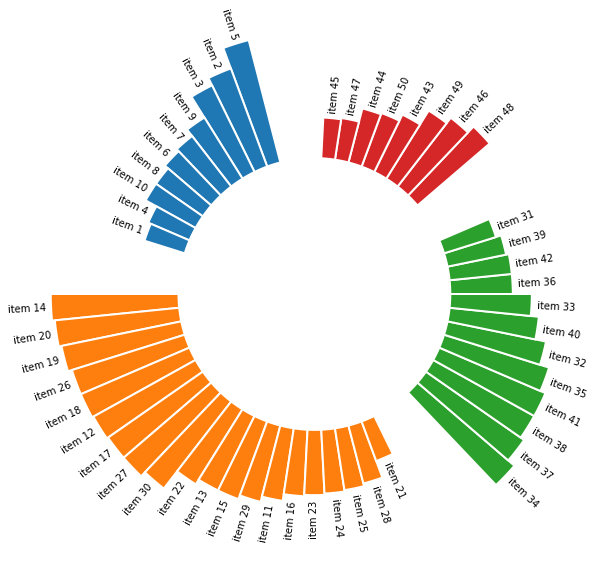## Circular barchart customization

Last but not least, it is highly advisable to add some customisation to your chart. Here we add group names (A, B, C and D), and we add a scale to help compare the sizes of the bars. Voila! The code is a bit long, but the result is quite worth it!

``````# All this part is like the code above
VALUES = df["value"].values
LABELS = df["name"].values
GROUP = df["group"].values

ANGLES_N = len(VALUES) + PAD * len(np.unique(GROUP))
ANGLES = np.linspace(0, 2 * np.pi, num=ANGLES_N, endpoint=False)
WIDTH = (2 * np.pi) / len(ANGLES)

offset = 0
IDXS = []
GROUPS_SIZE = [10, 20, 12, 8]
for size in GROUPS_SIZE:

fig, ax = plt.subplots(figsize=(20, 10), subplot_kw={"projection": "polar"})
ax.set_theta_offset(OFFSET)
ax.set_ylim(-100, 100)
ax.set_frame_on(False)
ax.xaxis.grid(False)
ax.yaxis.grid(False)
ax.set_xticks([])
ax.set_yticks([])

GROUPS_SIZE = [10, 20, 12, 8]
COLORS = [f"C{i}" for i, size in enumerate(GROUPS_SIZE) for _ in range(size)]

ax.bar(
ANGLES[IDXS], VALUES, width=WIDTH, color=COLORS,
edgecolor="white", linewidth=2
)

# Extra customization below here --------------------

# This iterates over the sizes of the groups adding reference
# lines and annotations.

offset = 0
for group, size in zip(["A", "B", "C", "D"], GROUPS_SIZE):
x1 = np.linspace(ANGLES[offset + PAD], ANGLES[offset + size + PAD - 1], num=50)
ax.plot(x1, [-5] * 50, color="#333333")

# Add text to indicate group
ax.text(
np.mean(x1), -20, group, color="#333333", fontsize=14,
fontweight="bold", ha="center", va="center"
)

# Add reference lines at 20, 40, 60, and 80
x2 = np.linspace(ANGLES[offset], ANGLES[offset + PAD - 1], num=50)
ax.plot(x2,  * 50, color="#bebebe", lw=0.8)
ax.plot(x2,  * 50, color="#bebebe", lw=0.8)
ax.plot(x2,  * 50, color="#bebebe", lw=0.8)
ax.plot(x2,  * 50, color="#bebebe", lw=0.8)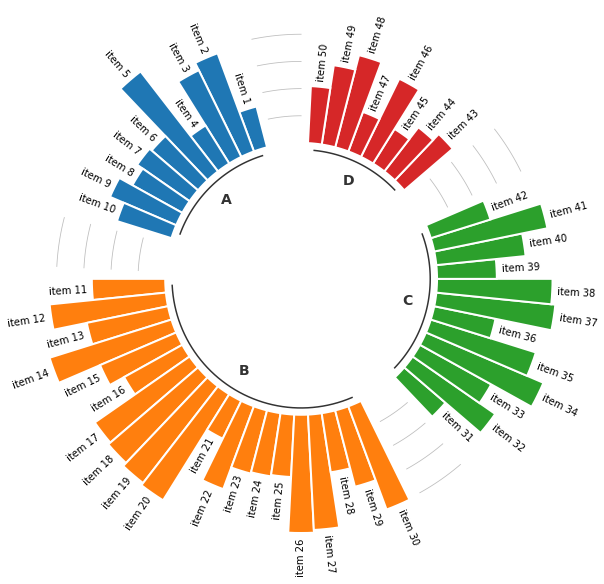👋 This document is a work by Yan Holtz. Any feedback is highly encouraged. You can fill an issue on Github, drop me a message onTwitter, or send an email pasting `yan.holtz.data` with `gmail.com`.